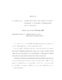## Matrix Factorizations, Triadic Matrices, and Modified Cholesky Factorizations for Optimization

 dc.contributor.advisor O'Leary, Dianne P en_US dc.contributor.author Fang, Haw-ren en_US dc.contributor.department Computer Science en_US dc.contributor.publisher Digital Repository at the University of Maryland en_US dc.contributor.publisher University of Maryland (College Park, Md.) en_US dc.date.accessioned 2006-09-12T06:00:27Z dc.date.available 2006-09-12T06:00:27Z dc.date.issued 2006-08-07 en_US dc.description.abstract This thesis focuses on the Cholesky-related factorizations of symmetric matrices and their application to Newton-type optimization. A matrix is called triadic if it has at most two nonzero off-diagonal elements in each column. Tridiagonal matrices are a special case of these. We prove that the triadic structure is preserved in the Cholesky-related factorizations We analyze its numerical stability and also present our perturbation analysis. Newton-like methods solve nonlinear programming problems whose objective function and constraint functions are twice continuously differentiable. At each iteration, a search direction is computed by solving a linear symmetric system Ax=b. When A is not positive definite, the computed search direction may not be a descent direction. Modified Newton methods add a perturbation E to A, so that A+E is positive definite, where E is symmetric positive semidefinite. We study the modified Newton methods in the literature, and develop other stable and efficient algorithms. One of them exploits the merits of triadic structure. We apply our modified Newton methods to the Euclidean distance matrix completion problem (EDMCP). Given n points in Euclidean space, the Euclidean distance matrix (EDM) is the real symmetric matrix with (i,j) entry being the square of the Euclidean distance between i-th and j-th points. Given a partial Euclidean distance matrix (i.e., some entries are not specified), the EDMCP is to find a EDM that includes the specified entries. We tackle the EDMCP by transforming it into a global optimization problem and applying modified Newton methods. We also develop a dimensional relaxation method for global minimization and test it on sample problems including protein structure prediction. en_US dc.format.extent 1162457 bytes dc.format.mimetype application/pdf dc.identifier.uri http://hdl.handle.net/1903/3891 dc.language.iso en_US dc.subject.pqcontrolled Computer Science en_US dc.subject.pqcontrolled Computer Science en_US dc.subject.pquncontrolled Cholesky Factorization en_US dc.subject.pquncontrolled Backward Error Analysis en_US dc.subject.pquncontrolled Tridiagonal Matrices en_US dc.subject.pquncontrolled Modified Newton Methods en_US dc.subject.pquncontrolled Interior Point Methods en_US dc.subject.pquncontrolled Distance Matrix Completion Problems en_US dc.title Matrix Factorizations, Triadic Matrices, and Modified Cholesky Factorizations for Optimization en_US dc.type Dissertation en_US
##### Original bundle
Now showing 1 - 1 of 1